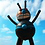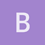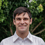This is an archived static version of the original phylobabble.org discussion site.

# Generating a set of trees that are as different from each other as possibleDan_Warren

Do any of you know of an existing method for constructing a set of trees that are as different from each other as possible? I’ve been thinking of trying a few things for possible inclusion in a future version of RWTY, and for some of them it would be nice to have a set of trees that are kind of like a Latin hypercube sampling of treespace. Obviously I could just generate a bunch of random trees and have some algorithm for picking a subset of them to maximize the sum of tree distances or something, but that could be quite time-consuming for large numbers of taxa. If there’s something more efficient, I’d like to know. I haven’t found anything with a bit of googling, and Rob L. suggested that someone here might have some idea of where to look.Brian_OMeara

I don’t know of such an algorithm. However, a sketch of one is to use quartets. Start with all three quartets for four species. To each of these, add a different quartet with a fifth species. Keep reiterating. Eventually you’ll have three trees with the same taxa but no quartets in common (I think: it’s possible some of the other quartets made in the process of adding trees together would overlap).Dan_Warren

That makes sense. Thanks, Brian!ematsen

@cwhidden and I have thought about related things, both the abstract question you pose about maximally distant topologies and also the implied question of if we can strategically pick a set of trees that will end up in various posterior modes. Nothing’s been written though.

On the other hand, random in large combinatorial spaces is pretty good! Chris reminded me of this nice result:

See https://arxiv.org/abs/1509.00669 for details.

So you are only picking up a factor of n^{1/6} by the hard work of finding the actual pair of maximally distant trees. How this would change if we were looking for more than two trees I couldn’t say.Dan_Warren

Oh hey, that’s great! It sounds like I may not need to muck with it at all, in that case.

Just to eyeball how much of an issue it is, I measured tree distances between a bunch of randomly chosen trees for data sets of different sizes. X axis is the number of randomly generated trees in a set, Y axis is the minimum tree distance between any pair of trees in the set, scaled by the maximum tree distance in the set. Obviously I don’t know how this compares to the performance of Brian’s suggestion, but given my intended use it looks like random trees are probably good enough.leomrtns

In your figure, for large trees it seems that the minimum is very close to the maximum, which can also mean that the distance you are using is not very discriminative.

My guess is that you are using the RF distance – and two random trees rarely have bipartitions in common, which explains the large RF. If that is what you want (no bipartitions in common), then you are fine. However there are other ways of defining “very different trees”: for instance if you move just one leave from one end to another of the tree, this will result in a large RF distance but the trees are still very similar in terms of the SPR distance or their maximum agreement subtree (MAST).

(I like Brian’s idea, BTW!)Dan_Warren

That’s a very good point. The plot looks pretty similar using SPR distances (below), but not quite as good.mathmomike

I don’t know if this result is helpful but here goes. For each n there is a set of (at least a constant times) n^2 binary phylogenetics trees, each of which is maximally distant from each other tree in that collection under the Robinson Foulds RF distance. In other words, no two of the Cn^2 trees share any non-trivial splits. My proof of this is non-constructive, but I’m pretty sure a (poly-time) construction should be possible.alexG

I was composing a post and phylobabble suggested me to look into this one tooIf ranked trees are of interest, this paper has an algorithm to produce trees as distant from a given tree as theoretically possible: https://doi.org/10.1101/2019.12.19.883603